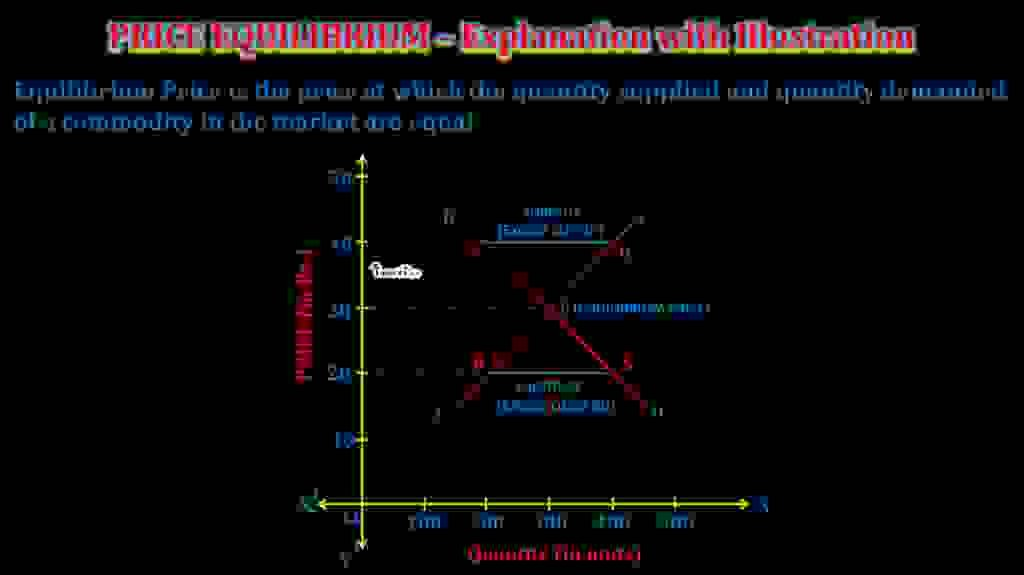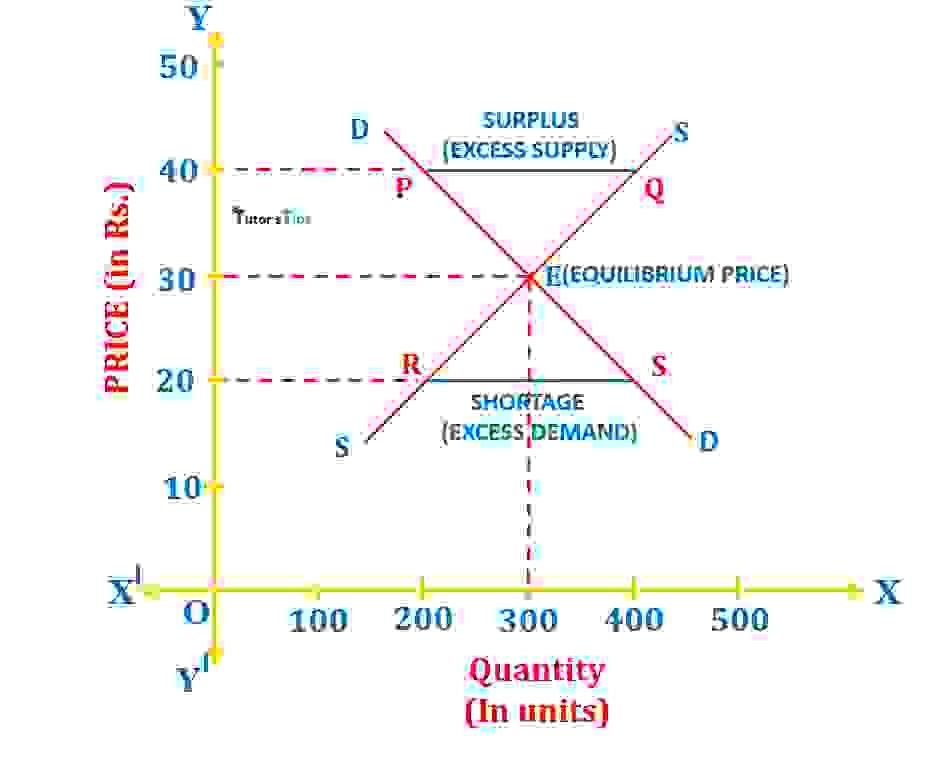# Market Equilibrium – Explanation with IllustrationMarket equilibrium is a situation where the demand for and supply of commodities are equal in the market corresponding to a particular price.

## Meaning of Market equilibrium:

Market equilibrium is defined as the situation when the demand of a commodity is equal to its supply corresponding to a specific price. Thus, in this situation, for a commodity,

Market Demand (DX ) = Market Supply (SX)

Hence, there is neither excess demand nor excess supply in the market. In such a situation, the prevailing price is known as Equilibrium price. And, quantity that is supplied or demanded is known as Equilibrium quantity.

Therefore, market equilibrium leads to:

Equilibrium Price: It is the price at which market demand of a commodity and its supply are equal.

Equilibrium Quantity: It is that quantity which corresponds to the equilibrium price.

## Basic Assumptions of Market Equilibrium:

1. Price and Quantity supplied are positively related to each other. Or, the supply curve of a commodity is upward sloping from left to right.

2. Price and Quantity demanded are inversely related to each other. It means the demand curve is downward sloping from left to right.

3. Forces of demand and supply operate freely in the market without government intervention.

## Determination of market equilibrium:

Under perfect competition, the market equilibrium is driven by market forces such as market demand and market supply.

### Market Demand :

Here, the market demand refers to the sum total of demand for a commodity by all the buyers in the market. It is represented by the market demand schedule and market demand curve.

### Market Supply :

And, the market supply refers to the total supply of a commodity by all sellers in the market. It is represented by the market supply schedule and the market supply curve.

### Market Equilibrium:

This situation is struck when the market demands of a commodity equal market supply. In other words, in this situation, there is no excess demand or excess supply in the market.

### Explanation with Illustration :

We can understand this with the following illustration:

Suppose, the demand and supply schedule for a commodity ‘X’ at different prices in the market is as follows:

 Price of commodity ‘X'(In Units) Quantity demanded of commodity ‘X'(In Units) Quantity Supplied of Commodity ‘X'(In Units) 50 100 500 40 200 400 30 300 300 20 400 200 10 500 100

The above table shows that at price Rs.50, the quantity demanded is 100 units whereas quantity supplied of commodity ‘X’ is 500 units. At this price, there is excess supply as the sellers have excess stock to offer. It means that sellers are willing to sell more than what consumers want to buy. This excessive supply forces the price to lower down in the market.

Suppose, the price falls to Rs 40, it leads to a rise in quantity demanded to 200 units and fall in supply to 400 units. Even at this price, the supply of commodity ‘X’ > its demand. Thus, the situation of excess supply prevails in the market. It again forces the price to fall, resulting in a new price at Rs 30. This fall in price leads to an increase in demand to 300 units and a decrease in supply to 300 units. Thus, Rs.30 is the equilibrium price as the quantity demanded and supplied are equal i.e.300 units. And the equilibrium quantity is 300 units.

#### Excess Demand:

Similarly, at any price below the equilibrium price, there would be excess demand. It means that there is a shortage of stock in the market as the quantity demanded by buyers is more than what sellers want to sell. At the equilibrium price, there is no excess demand and supply.

### Graphical Representation :Market Equilibrium

In fig, X-axis shows the quantity demanded by buyers and quantity supplied by sellers of commodity ‘X’ whereas Y-axis shows the different price levels. DD is the demand curve and SS is the supply curve. The point E is the equilibrium point where both curves intersect each other which implies Demand= Supply. This point shows that the equilibrium price is Rs.30 and the equilibrium quantity is 300 units.

If the price is more than equilibrium price i.e. Rs.40, it results in excess supply and shown by points P & Q where Supply is 400 units whereas Demand is only 200 units. The excess supply pressurizes the price to fall down in the market which leads to an increase in demand (movement along the curve from P to E)   and a decrease in supply (movement along the supply curve from Q to E). At point E, the excess supply is eliminated and results in demand = supply=300 units.

Likewise, if the price is less than the equilibrium price i.e. Rs 20, it results in excess demand and shown by points R & S where Supply is 200 units and Demand is 400 units. The excess demand forces the price to rise in the market which leads to an increase in supply(movement along the supply curve from R to E)  and a decrease in demand (movement along the supply curve from S to E). At point E, the excess demand is eliminated and results in demand = supply=300 units. Thus, the market is cleared and the equilibrium price is prevailing.

Thanks!!!

Please share with your friends

Comment if you have any question.

References:

Introductory Microeconomics – Class 11 – CBSE (2020-21)